# Gate Mock Test- 12: CS/IT

## 65 Questions MCQ Test GATE Computer Science Engineering(CSE) 2023 Mock Test Series | Gate Mock Test- 12: CS/IT

Description
Attempt Gate Mock Test- 12: CS/IT | 65 questions in 180 minutes | Mock test for GATE preparation | Free important questions MCQ to study GATE Computer Science Engineering(CSE) 2023 Mock Test Series for GATE Exam | Download free PDF with solutions
QUESTION: 1

### Santosh’s car gives 5 km more per litre of diesel when driven on the highway in comparison to city drive. On a recent trip, Santosh drove 30 km on the highway and 130 km in the city consuming a total of 15 litres of diesel in the process. How many km/litre does Santosh’s car run in the city?

Solution: Let the mileage of Santosh's car be n km/litre of diesel when driven in the city and (n+5) km/litre when driven on the highway. Translating the given information in to an equation, we can write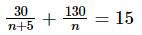⇒ 3n2 + 17n – 130 = 0

Which gives n = 10 or n = -13/3

Hence we can say that Santosh's car runs 10 km/litre in the city.

QUESTION: 2

### Choose the option which is opposite in meaning to RELINQUISH.

Solution: ‘Relinquish’ refers to surrender or give up whereas ‘possess’ refers to have or own something.
QUESTION: 3

### Choose the best alternative which can be substituted for the given sentence”: “A person difficult to please”

Solution: ‘Fastidious’ is someone who is excessively particular demanding or hard to please.
QUESTION: 4

Two solutions of alcohol A and B were mixed to obtain 20 litres of new solution C. Before they were mixed, the first solution A contained 1.6 litres of alcohol while the second solution B contained 1.2 litres of alcohol. Before mixing if the percentage of alcohol in the first solution A was twice that in the second B, what was the volume of the first solution A before mixing?

Solution: Let the volume of the first A in the mixture be "x′′ "Itres, the volume of the second B in the mixture must be (20 − x)

% of alcohalin A=(1.6 / x) × 100

% of alcohalin B=(1.2 / 20 − x) × 100

(1.6 / x) × 100 = 2 × (1.2 / 20 − x) ×1 00

16 − 0.8x = 1.2x

x = 8 litres

QUESTION: 5

In this question, out of the four alternatives, choose the option which is closest in meaning to FURORE.

Solution: ‘Furore’ refers to an outbreak of public anger or excitement.
QUESTION: 6

Find the pair of letters which will come in blank spaces marked as ‘?’.

V W X Y E D C B R S T ? ?

Solution:

A careful look at the alphabets in the given series shows a pattern

VWXY – EDCB RST? etc

∨ ↔ E; W ↔ D; X ↔C; Y ↔ B i.e. 5th from end corresponds to a* from the beginning etc. It is a cluster of 4 consecutive letters which is taken at a time, "Therefore, the next 4 letters will be RSTU-IHGF etc.

Hence the last 2 letters will be U and I i.e. option (b).

QUESTION: 7

We are given a square of side 22 cm. A circle of maximum possible diameter is inscribed in this square. If a point is chosen at random inside the square, then the probability that it will lie inside the circle is ____________.

Solution:

Required probability = Area of the circle / Area of the square

Biggest possible circle that can be inscribed in the given square would, be touching all the four sides of the square internally implying that the diameter of this circle = side of the square = 22cm

Required probability =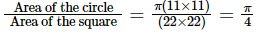= 0.785

QUESTION: 8

Based on the given statements, select the most appropriate option to solve the question.

Sheetal wants to sell her bicycle at either a profit of K% or a loss of K%. What is the value of K?

Statement 1: Difference between the amount Sheetal gets in the 2 cases is ₹ 2560

Statement 2: If Sheetal’s profit is Rs K, her profit in percentage is 7.5%.

Solution:

Let us assume k = K / 100 and the cost price = C

Based on SI, we can write C × (1 + K / 100) − C × (1 − K / 100) = 2560

i.e. 2CK / 100 = 2560 or Ck = 1280 which does not give the value of k or K. Hence Statement 1 is NOT sufficient.

Based onS2, C × 0.075 = K which gives C = 40K / 3 = 4000k / 3 which will NOT give the value of k or K.

When we combine the information given in both the statements, we will be able to find C as well as k or K. Hence option (c) is the correct option.

*Answer can only contain numeric values
QUESTION: 9

Triangles ABC and CDE have a common vertex C with side AB of triangle ABC being parallel to side DE of triangle CDE. If length of side AB = 4 cm and length of side DE = 10 cm and perpendicular distance between sides AB and DE is 9.8 cm, then the sum of areas of triangle ABC and triangle CDE is _________ cm2.

Solution: The answer is in between 40 and 41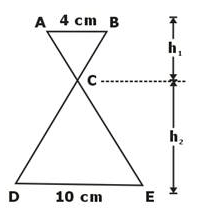Given AB‖DE

⇒ ∠B = ∠D (Alternate angles)

and ∠A = ∠E (Alternate angles)

△ABC − ΔEDC (AAA similarity)

⇒ h1 / h2 = AB / DE = 410 = 25

and h1 + h2 = 9.8cm (given)

h1 = 2.8cm and h2 = 7cm

Area of ΔABC = 12 × 4 × 2.8 = 5.6cm2

Area of ΔEDC = 12 × 10 × 7 = 35cm2

∴ Sum of areas of ΔABC and ΔEDC = 40.6cm2

QUESTION: 10

Interior angles of a polygon with 8 sides are in arithmetic progression. If the smallest interior angle measures 100° then find the largest interior angle?

Solution:

If 100 is the smallest interior angle of the polygon i.e. Octagon, this gives the largest exterior angle = 180 100= 80

The sum of exterior angles of a convex polygon = 360

since the interior angles form an increasing arithmetic progression, the exterior angles will form a decreasing arithmetic progression, let us say with common difference 'd "

360 = 80 + (80 + d) + (80 + 2d)……8 terms

= 82[2 × 80 + (8 − 1)d] = 360 which gives d = −10

Using d=−10, we get the smallest exterior angle =80 + (8−1) × (−10) = 10 leading to the largest interior angle =180 − 10 = 170

Note: formula used for sum of terms of an AP is given by

Sn = n2[2a + (n − 1)d] where 'a' is the first term and 'd' is the common difference.

Alternatively,

Sum of all the interior angles of a polygon = (n − 2)180

⇒ a + (a + d) +……8 terms = (8 − 2)180∘

⇒ 82(100 × 2 + 7d) = 1080

⇒ d = 10

∴ Largest angle = a + 7d = 100 + 7(10) = 170

QUESTION: 11

Consider the following problems.

1. longest common subsequence

2. Optimal Binary search tree

3. Fractional knapsack problem

4. Matrix chain multiplication

Which of the above problems can be solved using dynamic programming?

Solution: Longest common subsequence, longest increasing subsequence, sum of subsets, optimal BST, matrix chain multiplication, Travelling salesperson, Balanced partition, Fibonacci sequence, Multistage graph problems , 0/1 knapsack are solved by using dynamic programming.
QUESTION: 12

Suppose that the minimum spanning tree of the following edge weighted graph contains the edges with weights x, y and z.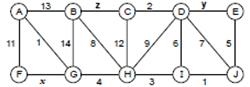What is the maximum value of x+y+z?

Solution:

x ≤ 11

y ≤ 6

z ≤ 8

QUESTION: 13

Which of the following standard algorithm is not an greedy algorithm?

Solution: Bellman ford shortest path algorithm is example of dynamic programming.
QUESTION: 14

For the sequence

500, 535, 512, 721, 436, 611, 624, 632, 643

Lexicographic sort gives time complexity of:

Solution: T(n) = O(m × n) = O(3 × 9) = O(27)

Where m are the number of digits on each element of sequence

n : number of elements in sequence.

QUESTION: 15

Consider the following SDT

G→A-T{G.x=A.x-T.x}

A→Ea{A.x=E.x2}

EEb{E1.x=1+E2.x}

E→ξ{E.x=-1}

T→Eb(T.x=Ex-2}

If above SDT uses L- attributed definition then what is the value of an attribute x at root after evaluation for an input string “ba-bb”.

Solution: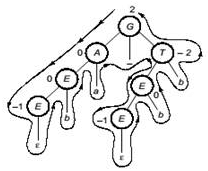In the above dependency graph, 2 is the value at G after the evaluation.

QUESTION: 16

Match the following: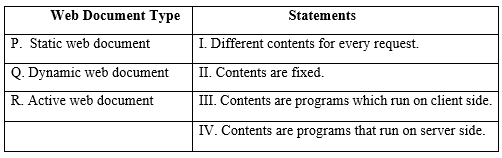Solution: Active web documents are the programs that run on the client side. The best example of active web documents is animated graphics on the screen which helps in interaction with clients.

While for static documents as the name suggests, contents are static.

QUESTION: 17

While opening a TCP connection, the initial sequence number is to be derived using a time-of-day (ToD) clock that keeps running even when the host is down. The low order 32 bits of the counter of the ToD clock is to be used for the initial sequence numbers. The clock counters increments once per millisecond. The maximum packet lifetime is given to be 64s.

Which one of the choices given below is closest to the minimum permissible rate at which sequence numbers used for packets of a connection can increase?

Solution:

The maximum packet lifetime is given to be 64 seconds in the question.

Thus, a sequence number increments after every 64 seconds.

So, minimum permissible rate = 1 / 64 = 0.015 per second.

QUESTION: 18

Both hosts and routers are TCP/IP protocol software. However, routers do not use protocol from all layers. The layer for which protocol software is not needed by a router is

Solution: The features that stood out during the research, which led to making the TCP/IP reference model were:
• Support for a flexible architecture. Adding more machines to a network was easy.
• The network was robust, and connections remained intact until the source and destination machines were functioning.

The overall idea was to allow one application on one computer to talk to(send data packets) another application running on a different computer.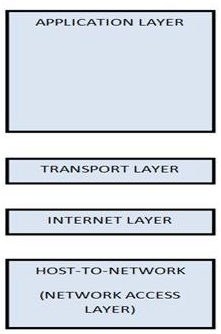QUESTION: 19

If the instruction length in a system is 32 bits and memory has 32 words. Then find the 1 address instructions if there are 42 address instructions’.

Solution: Number of 1 address instructions =(25 – 4) × 25 = 896.
QUESTION: 20

Consider execution of 100 instructions on a 5 stage pipeline. Let P be the probability of an instruction being a branch. What must be the value of P such that speed up is atleast 4?

(Assume each stage takes 1 cycle to perform it’s task and branch is predicted on fourth stage of the pipeline)

Solution: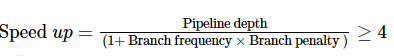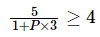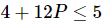12P ≤ 1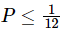= 0.08

QUESTION: 21

What is the output of the following C code?

#include

#include

void main()

{

int index;

for(index=1; index<=5;i++)

{

printf("%d",index);

if(i == 3)

continue;

}

}

Solution: Here continue is the last line. So, it will not skip any number.
QUESTION: 22

Consider the following code

int DO(char *gate)

{

char *gate1 = gate;

char *gate2 = gate + strlen (gate) – 1;

while (gate1 < =gate2)

{

if (*gate1 ++! = *gate2 --)

Return 0;

}

return 1;

}

What is the functionality of the above function Do ()?

Solution: Here two pointers are used: gate 1 and gate 2. One pointer points to the beginning and other to the end. Loop is a set up that compares the characters pointed by these two pointers. If the characters do not match then it’s not a palindrome. It returns 1 for both even and odd palindromes.
QUESTION: 23

Consider the following code for inorder traversal of a binary tree.

Void traversal (node *root)

{

if (root ==0)

{

Print f(“empty tree”);

}

else

{

itraversal (root left);

printf("%d\n”, root data);

Iiraversal (root right);

}

}

Which of the following is true about the above snippet of code.

Solution: “Empty tree” will be printed (N+1) times in addition to inorder traversal. It is so because, if root is zero, the control has to return, but it instead just prints “empty tree” (N+1) times, because for a binary tree with N nodes, there are (N+1) empty nodes.
QUESTION: 24

R{a,b,c} and S{x,y,z} are two relations in which x is a foreign key referring to the primary key of relation R.

Consider the following operations. Which among these operations may violate the referential integrity constraint?

Solution: x is a foreign key referring to the primary key of the relation R. If we delete anything from R, it may so happen that there is a value in S linked to the deleted value. Or if we insert something in S, it may not be present in relation R.
QUESTION: 25

Suppose that we have the following three tuples in a legal instance of a relation schema S with three attributes ABC (listed in order): (1,2,3), (4,2,3), and (5,3,3). Which of the following dependencies can you infer does not hold over schema S?

Solution: BC→ A does not hold over S (look at the tuples (1,2,3) and (4,2,3)). The other tuples hold over S.
QUESTION: 26

Number of binary trees formed with 5 nodes are

Solution: Possible number of binary trees formed when n number of nodes are given is 2nCn / n+1 so in this case the number of nodes are 5 so

Number of binary trees possible are = 10C5 / 6 = 10!/ 5! * 5! * 6 → 42

Hence option D is the correct answer

Total number of different Binary tree of size

5 : 14 + 5 + 4 + 5 + 14 = 42

QUESTION: 27

The Boolean function f implemented in the figure using two input multiplexers is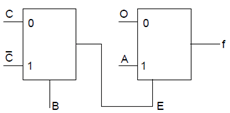Solution: f = E.A

E =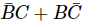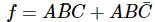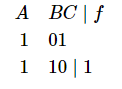QUESTION: 28

Consider a complete undirected graph A with 6 vertices. All the vertices are labelled. What is the number of distinct cycles of length 4 in this graph?

Solution: We can pick 4 vertices from 6 in 6C4 ways i.e. 15. Now, there can be 3 distinct cycles from 4 vertices

A--------B

| |

| |

| |

C--------D

Distinct cycles = ABC , ACD , BCD

Hence 15×3 = 45 is the answer.

QUESTION: 29

The number of colours required to properly colour the vertices of every planar graph is

Solution: According to the 4-colour theorem, the vertices of every planar graph can be coloured with at most 4 colours so that no two adjacent vertices receive the same colour.

Hence, Option (c) 4 is the correct choice.

QUESTION: 30

While opening a TCP connection, the initial sequence number is to be derived using a time-of-day (ToD) clock that keeps running even when the host is down. The low order 32 bits of the counter of the ToD clock is to be used for the initial sequence numbers. The clock counters increments once per millisecond. The maximum packet lifetime is given to be 64s.

Which one of the choices given below is closest to the minimum permissible rate at which sequence numbers used for packets of a connection can increase?

Solution:

The maximum packet lifetime is given to be 64 seconds in the question.

Thus, a sequence number increments after every 64 seconds.

So, minimum permissible rate = 1 / 64 = 0.015 per second.

QUESTION: 31

Consider the following SDT :

E → E + T {E.val = E.val - T.val , Printf(E.val)}

E → T {E.val = T.val+1}

T → T*F {T.val = T.val + F.val}

T → F {T.val = 2*F.val}

F → id {F.val = id}

What will be printed when the provided input is : 3*4*5+7 _________.

Solution: The parse tree is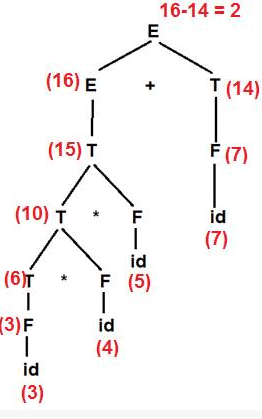QUESTION: 32

During intermediate code generation, we use 3 - address code in which we have 3 forms available : Quadruples, Triples and Indirect Triples. Now consider the below statements :

S1 : In Quadruples, statements can be moved around

S2 : In Triples, space is not wasted.

S3 : In Indirect Triples, space is not wasted but access time increases.

Which is correct?

Solution:

S1 : It is true, in quadruples, statements can be moved.

S2 : In Triples, space is not wasted because the result field is not used.

S3 : Here two memory accesses are required, that’s why access time is more.

QUESTION: 33

In Prim's algorithm, we use decrease key operation. What is the time complexity of this decrease key operation in case of Prim's Algorithm :

Solution: In Prim's algorithm, heaps of vertices are created and decrease key operation is performed on the heap elements which takes O(logn) time. Decrease key operation is performed to change the value of distance in every iteration.
QUESTION: 34

Consider double hashing where the hash function is HF(key) = (HF1(key) + iHF2(key))mod7

HF1(key) = Key mod 7

HF2(key) = R - (Key mod R)

where R is the maximum prime number less than the table size.

The following keys are being inserted into the table :

76 , 93 , 40 , 47 , 10 , 55

Find the number of times the second hash function needs to be used while hashing the keys _______.

Solution: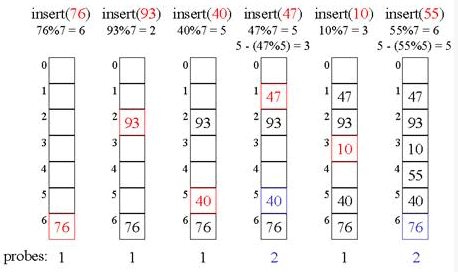Hence 2 times a second hash function is used.

QUESTION: 35

Consider the following weighted graph :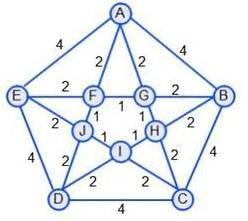Find the weight of Minimum Cost spanning tree ____________.

Solution:

There can be multiple spanning trees possible but all the spanning trees will have the same weight.

The edges in MST will be : AF , EF , FG , BG , FJ , JP , GH , IH and CH.

Total weight = 14

QUESTION: 36

Consider an ordered file with 1,00,000 records stored on a disk using un-spanned file organization. Block size is 2048 bytes and record length 256 bytes. If primary indexing is used over a field of size 10 bytes and block pointer size is 6 bytes. Then a number of block access is required to search for a record.

Solution:

Number of records per block = 2048/256 =8

Size of each index entry = 10+6 = 16.

number of entries per block =2048/16 = 128

Total number of indexes = total number of blocks in file = 100000/8 =12500

So, number of index blocks = ceil(12500/128) = 98

Total number of block access = 1+ceil(log298) =1+7 = 8.

QUESTION: 37

Which of the following is the primary goal of the Operating System when programmed for the Technical workplaces?

Solution:

Convenience is the primary goal when programming is done for workplaces and Efficiency is the primary goal when programmed for users.

QUESTION: 38

Assume that a sliding window protocol is designed for a 1Mbps point to point link to destination, which has a one-way latency of 1.25 sec. Assuming that each frame carries 1 kB of data, what is the minimum number of bits needed for the sequence number?

Solution:

Tp = 1.25 sec

Tt = Data/Bandwidth = 1024 x 8 / 106 = 8.192 ms

window size should be = 1 + 2a, for having maximum efficiency = 306.17

So, number of bits for sequence number = log2(306.17) = 9 bits

QUESTION: 39

On a system using paging and segmentation, the virtual address space consists of up to 8 segments where each segment can be up to bytes long. The hardware pages each segment into 256 bytes pages. Bits in the virtual address are- Page number

Solution:

with 256 = 28 byte pages, a 229 byte segment can have 229/28=221 pages. Thus, 21 bits are needed to specify the page number.

QUESTION: 40

What is the value of X+ Y if X be the maximum number of nodes and Y be the maximum number of keys present in the B tree with order P=5 and level=4__________ where P is the Max no of pointers.

Solution:

Here, No of Level = 5 and P = 4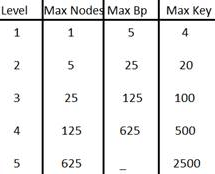Hence, X = 625 + 125 + 25 + 5 + 1= 781

Y = 4 + 20 + 100 + 500 + 2500 = 3124

X + Y = 3905

QUESTION: 41

The orange box in the below figure consists of a minimum complexity circuit that uses only AND, OR and NOT gates. The function f(x,y,z) = 1 where any of the following conditions are satisfied :

• x is not equal to y and z is grounded
• y is equal to z , and x is set low
• x is equal to y , y is equal to z but x not equal to z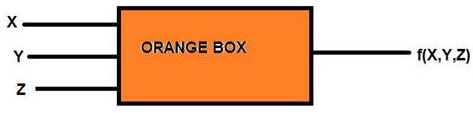Then which of the following equations leads to correct design for the minimum complexity circuit:

Solution:

Construct the truth table using the above rules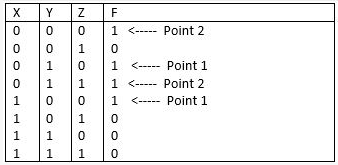Point 3 is not logically correct, hence no value set high in the truth table against point3.

Now use K - Map to realize the minimized Boolean expression :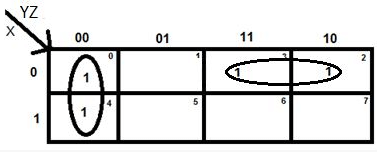The required expression is : y'z' + x'y (option c)

QUESTION: 42

Consider a file of 8192 records. Each record is 4bytes long and its key field is of size 6 bytes. The file is ordered on a key field, and the file organization is unspanned. The file is stored in a file system with block size is 512 bytes, and the size of a block pointer is 10bytes. If the primary index is built on the key field of the file, and a multilevel index scheme is used to store the primary index, the number of second level blocks in the multilevel index are-

Solution:

DB size 512

record size 16

no of record possible in disk block 512/16 = 32 records/block

no of Disk block present in DB file 8192/32 = 256

So a primary index is used which is called a sparse index. In this index we make entries of each disk block but not of every record.!

so at first level we have = 256/(index file size )

now wt is index file : with the help of block pointer and search we locate records so index file size = 512/(6+10) = 512/16 = 32 (records per index )

so at first level we have 256/32 = 8 index blocks

and hence multilevel index is used so on the second level we have only 1 index block.

QUESTION: 43

What is the minimum space utilization for a B+ tree index in percent?

Solution:

By the definition of a B+ tree, each index page, except for the root, has at least d and at most 2d key entries. Therefore—with the exception of the root—the minimum space utilization guaranteed by a B+ tree index is 50 percent.

QUESTION: 44

Assume a sequence of memory accesses is made that have a high degree of temporal locality, which one of these cache would be likely to have the best performance?

Solution:

To have high temporal locality the data should be retained longer. Temporal locality means that a data accessed has a high probability of being accessed again. So to maximize temporal locality we have to ensure that the data is not replaced with another block. And comparing the given cache configurations, 4-way set associative cache with 2 byte blocks is the best choice.

QUESTION: 45

Substitution of values for names (whose values are constants) is done in

Solution:

Constant folding is the process of recognizing and evaluating constant expressions at compile time rather than computing them at runtime.

QUESTION: 46

For which of the following graphs, Topological ordering is not possible?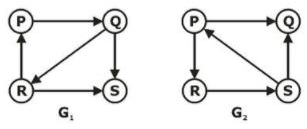Solution:

Topological ordering is possible only when graph do not have directed cycle. But in graph G1 it contains a directed cycle PQR. So, we cannot find Topological ordering.

QUESTION: 47

Consider the Following IEEE 32 bit representation:

0 01111110 10100000000000000000000

Its decimal equivalent is ___________ (Upto 4 decimal places)

Solution:

The IEEE format for 32 bit floating point arithmetic is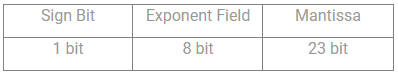Sign Bit= 0 (positive sign)

Biased Exponent= 01111110(126)

It is less than bias then it is negative

Actual Exponent= 126- 127= -1

So it can be represented using normalised form as:

1.101 * 2-1

è 0.1101

è 0.8125

QUESTION: 48

By adding (36)7, (67)8, (98)10 and (34)5 these four numbers with different bases, what will be the result in Base 9?

Solution:

(36)7= 7×3+6= (27)10

(67)8=8×6+7= (55)10

(98)10=(98)10

(34)5= 3×5+4=(19)10

∴ 27+55+98+19=(199)10

=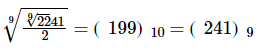= 241

QUESTION: 49

Which of the following flip-flops is used to avoid race around problems?

Solution:

Master-Slave flip flop is free used to avoid the race around condition.

QUESTION: 50

Which switching technique is used in the telephone network?

Solution:

Circuit switching technique is used in telephone networks. In this technique a dedicated network path is established for communication, for this, when two persons talking on telephone through one network path, no one else can use that path even if no talking (means no signal transfer through that path) is done.

QUESTION: 51

Which of the following statements about normal forms is false?

Solution:

Lossless, dependency preserving decomposition into BCNF is always possible,

Lossless, dependency preserving decomposition into BCNF is always not possible. All other statements are correct.

QUESTION: 52

Consider the following 2 × 2 matrix A where two elements are unknown and are marked by a and b. The eigenvalues of this matrix are -1 and 7. What are the values of a and b?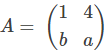Solution:

The character equation for given matrix is

| 1-λ 4 | = 0

| b a-λ|

(1-λ) × (a-λ) - 4b = 0

Putting λ = -1,

⇒ (1 - (-1)) × (a - (-1)) - 4b = 0

⇒ 2a + 2 - 4b = 0

⇒ 2b - a = 1

Putting λ = 7,

⇒ (1 - 7) × (a - 7) - 4b = 0

⇒ -6a + 42 - 4b = 0

⇒ 2b + 3a = 21

Solving the above two equations, we get

a = 5, b = 3

QUESTION: 53

A 100 MHz processor is connected to a device through a DMA controller. Assume that the initial set-up of DMA takes 100 clock cycles. The device has a transfer rate of 200 Kbytes/sec and average block size transferred is 4 KB. What fraction (in %) of the processor time is consumed by the device.

Solution:

Cycle time of processor =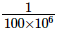= 10ns

Processor time = 100 x 10ns = 1ߎs

Data transfer time =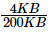= 20ms

% of processor time consumed by the device

=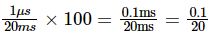= 0.005

QUESTION: 54

Consider building a CSMA/CD network running at 10Mbps over a cable with no repeaters. If the signal speed in the cable is 106 km/sec and minimum frame size is 1500 bytes then what is the cable length in km ?

Solution:

In CSMA/CO, TT = 2PT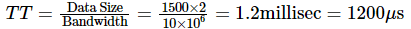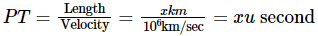So, 1200 = 2 X x

⇒ x = 600km

QUESTION: 55

Suppose the maximum Sequence no which is possible with Go-Back N, Stop and Wait and Selective repeat protocol is k. What is the size of the Sender window for each protocol respectively?

Solution:

Sender window size for stop and wait protocol is always 1.

And, for going back it is on less than no of sequences which are possible. if N be the total no of sequences possible, than, K = N - 1, i.e., Size of sender’s window = K

For Selective repeat protocol, No of sequences which are possible= (Sender window size + Receiver window Size) / 2

Also, Sender Window Size = Receiver Window Size

Then, No of sequences Possible = Sender window Size

Hence, Sender Window Size = K + 1

QUESTION: 56

Two concurrent processes P1 and P2 want to use resources R1 and R2 in a mutually exclusive manner. Initially, R1 and R2 are free. The programs executed by the two processes are given below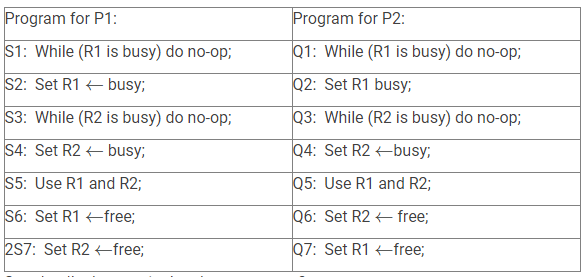Can deadlock occur in the above program?

Solution:

Yes deadlock is not possible because at least one process can enter into the critical section as it is mentioned in the question that initially both the resources are free. Thus, at least one process will execute in a critical section at a time and the resources used by that process will be set to busy & therefore another process will have to wait to use the critical section.

QUESTION: 57

Given the relations employee (name, salary, deptno), and department (deptno, deptname, address)

Which of the following queries cannot be expressed using the basic relational algebra operations (σ,π,×, ,∪,∩,−)?

Solution:

There are six basic relational algebra, they are selection(σ), projection(π), Cartesian product(×), set union(∪), set difference(−), rename. We can not omit these six fundamental operators. Among these intersections, division, natural join are defined under this six operators, but aggregation is not possible with algebra operations, so we cannot express the basic algebra operations in sum of all the employee salaries.

QUESTION: 58

The mechanism involves in direct searching is:

Solution:

Hashing is one way to enable security during the process of message transmission when the message is intended for a particular recipient only.

QUESTION: 59

Symbol table is accessed during ___ phase of a compiler.

Solution:

During lexical analysis, attribute information of the token is updated in the symbol table.

During semantic analysis, type of identifiers (tokens) is updated in the symbol table.

⇒ Option c is correct.

QUESTION: 60

Consider the following recursive c function: PROGRAMMING

void ABC (int i)

{

if(i<1)

return;

ABC(i-2);

ABC(i-4);

printf(“%d”, i);

}.

If the ABC (6) function is being called in main () then how many times will the ABC() function be invoked before returning to the main()?

Solution:

ABC(0) = 1

ABC(-1) = 1(itself).

ABC(1) = ABC(-1)+ABC(-3)+1 = 3

In general ,

ABC(i) = ABC(i-2) + ABC(i-4) + 1.

ABC(6) = ABC(4) + ABC(2) + 1

ABC(2) = ABC(0) + ABC(-2) + 1

= 1 + 1 + 1 = 3

ABC(4) = ABC(2) + AB(0) + 1

= 3 + 1 + 1 =5

ABC(6) = 5 + 3 + 1

= 9.

QUESTION: 61

Assume an instruction set that uses a fixed 16-bit instruction length. Operand specifiers are 6 bits in length. There are K two-operand instructions and L zero-operand instructions. What is the maximum number of one-operand instructions that can be supported?

Solution:

Suppose X be the no of One Address Instruction. The feasibility of K two address, X one address and L zero address instruction requires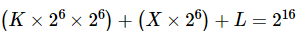Calculating for X, we get

X =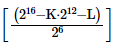QUESTION: 62

Which of the following statements is false?

Solution:

(a) TRUE. Since BFS finds paths using the fewest number of edges, the BFS depth of any vertex is at least as small as the DFS depth of the same vertex. Thus, the DFS tree has a greater or equal depth.

(b) FALSE. Even if no two edges have the same weight, there could be two paths with the same weight. For example, there could be two paths from s to t with lengths 3 + 5 = 8 and 2 + 6 = 8. These paths have the same length (8) even though the edges (2, 3, 5, 6) are all distinct.

(c) TRUE. It is true that the absence of back edges with respect to a DFS tree implies that the graph is acyclic.

(d) TRUE. With an adjacency matrix representation, visiting each vertex takes O(V ) time, as we must check all N possible outgoing edges in the adjacency matrix. Thus, BFS will take O(V 2 ) time using an adjacency matrix.

QUESTION: 63

Horizontal microprogramming

Solution:

In horizontal microprogramming the instruction size is less as compared to vertical microprogramming. So, there is no need for decoding. But, one bit is used for all control signals to execute the microinstruction. If the bit is set to ‘1’ the control signal field is activated. If the bit is set to ‘0’ the control signal field is deactivated. Thus, option D. is correct. Microprogramming: A method of accomplishing the control unit function by describing the steps in that function as a sequence of register-transfer level operations that are much more elementary than instructions. In this method of designing and building a control unit, an additional memory, commonly called a microprogram store, contains a sequence of microinstructions. A number of microinstructions will be required to carry out an ordinary machine instruction, thus the microprogram store should be faster – have a shorter cycle time – than the normal fast memory.

QUESTION: 64

Consider the following grammar with corresponding synthesized attributes.

F→L{F. yal =L. yal }

L→LB{ L. len =L1 . len +1, L. val =L1 . val +2 -Llen × B. val }

L→B{L. len =1,L. val =B. val/ 2}

B→0{B. yal =0}

B→1{B, val =1}

If “F.val” gives the value of the binary fraction generated by F in the above grammar then that will be the value of F.val on input .101?

Solution:

Given grammar

F→ .L

L→ LB

L →B

B →0

B →1

Input string .101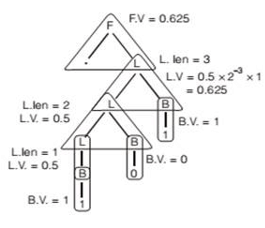QUESTION: 65

Which of the following is NOT an advantage of using shared, dynamically linked libraries as opposed to using statically linked libraries?

Solution:

In Non-Shared (static) libraries, since library code is connected at compile time, the final executable has no dependencies on the the library at run time i.e. no additional run-time loading costs, it means that you don’t need to carry along a copy of the library that is being used and you have everything under your control and there is no dependency.Use Code STAYHOME200 and get INR 200 additional OFF Use Coupon Code